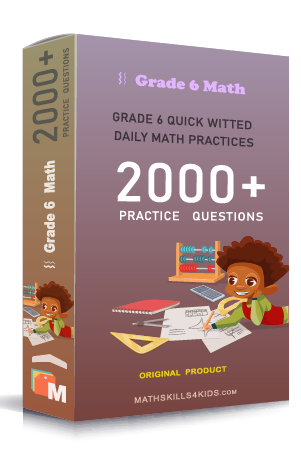# Grade 6 Decimals worksheets with solutions

Subject
Math

Resource Type
Worksheets, Printables, Homeschool

Standards
CCSS 6.NS.C.6c

Format
PDF (13.4 MB | 18 pages → Exercises + Solutions)

• ### -15% OFF Over \$100

Promo Code: TOPLA

After Purchase

\$2.35

This package allows you to practice the following skills

Writing decimal numbers from illustrated models

Understanding decimal place value

Writing word names for decimal numbers

Putting decimal numbers in orders

Practicing inequalities with decimals (>, <, and =)

Rounding decimals

Rounding decimals to whole numbers

Writing decimal on a number line

Repeating decimal numbers

This product is a part of the Mega Pack
* Grade 6 quick-witted daily math practices *